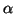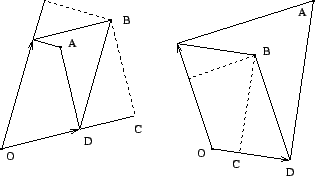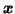Next: Nonlinear Independent Factor Analysis Up: Update Rules Previous: Stabilising the Fixed-Point Update

### Using Additional Information for Updating Sources.

With sources, it is possible to measure and compensate some of the correlated effects in the updates. Recall that the Jacobian matrix of the outputof the network w.r.t. the sources was computed when taking into account the effects of multiple paths of propagating the values of sources. This will be used to compensate the assumption of independent updates, in addition to the learning rates.

Suppose we have two sources whose effect on outputs are positively correlated. Assuming the effects independent means that the step will be too large and the actual step size should be less than what the Newton iteration suggests. This can be detected from computing the change resulting in the outputs and projecting it back for each source independently to see how much each source alone should change to produce the same change in the outputs. The difference between the change of one source in the update and change resulting from all the updates can then be used to adjust the step sizes in the update.Two examples of correction are depicted in Fig. 5. The left hand side graph shows a case where the effects of sources on the outputs are positively correlated and the right hand side graph has negatively correlated effects. Current output of the network is in the origin O and the minimum of the cost function is in point A. Black arrows show where the output would move if the sources were minimised independently. The combined updates would then take the output to point B.

As the effects of sources onare correlated, point B, the resulting overall change in, differs from point A. Projecting the point B back to the sources, comparison between the resulting step size C and the desired step size D can be used for adjusting the step size. The new step size on the source would be D/C times the original. With positively correlated effects the adjusting factor D/C is less then one, but with negatively correlated sources it is greater than one. For the sake of stability, the corrected step is restricted to be at most twice the original.Next: Nonlinear Independent Factor Analysis Up: Update Rules Previous: Stabilising the Fixed-Point Update
Harri Lappalainen
2000-03-03